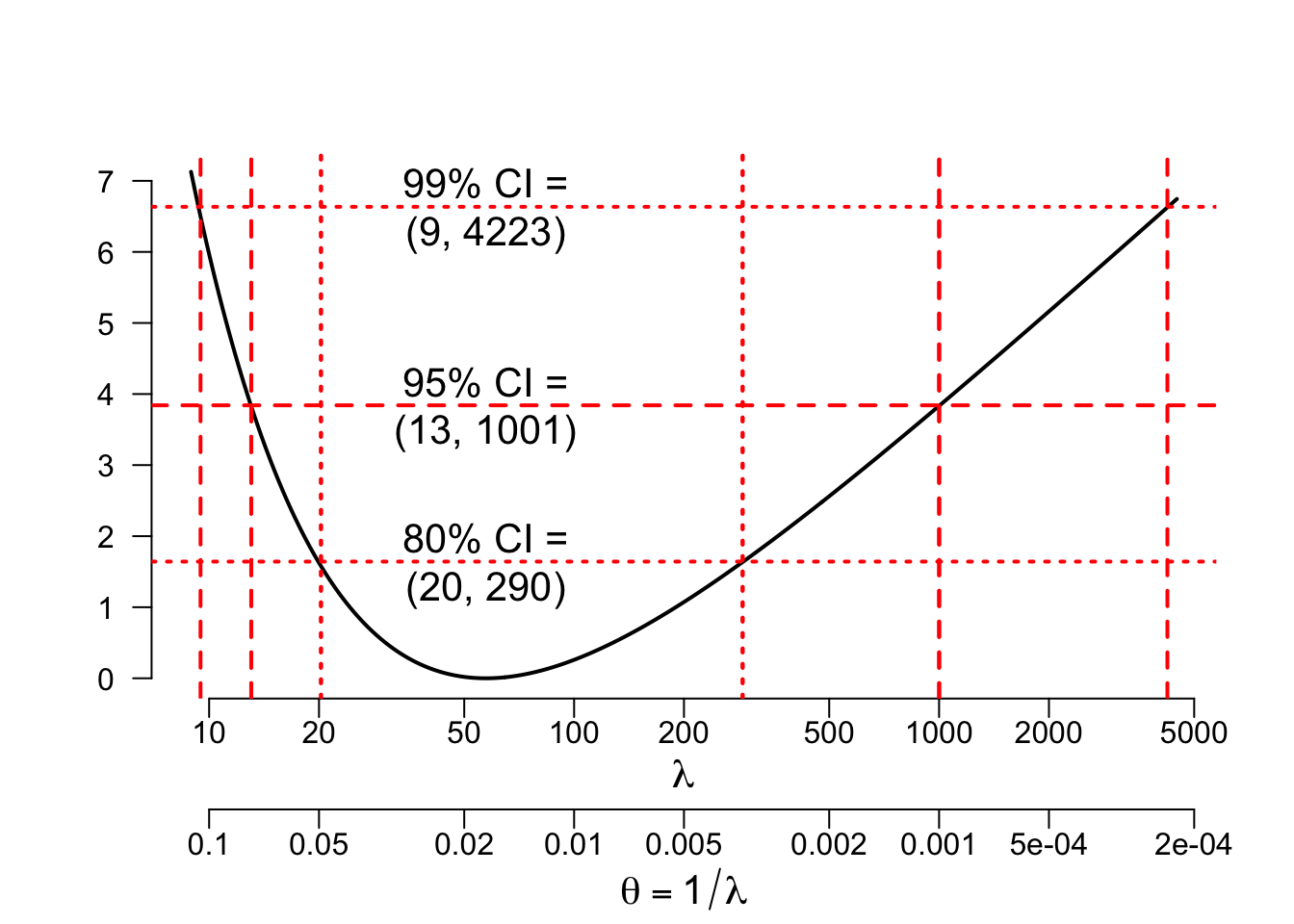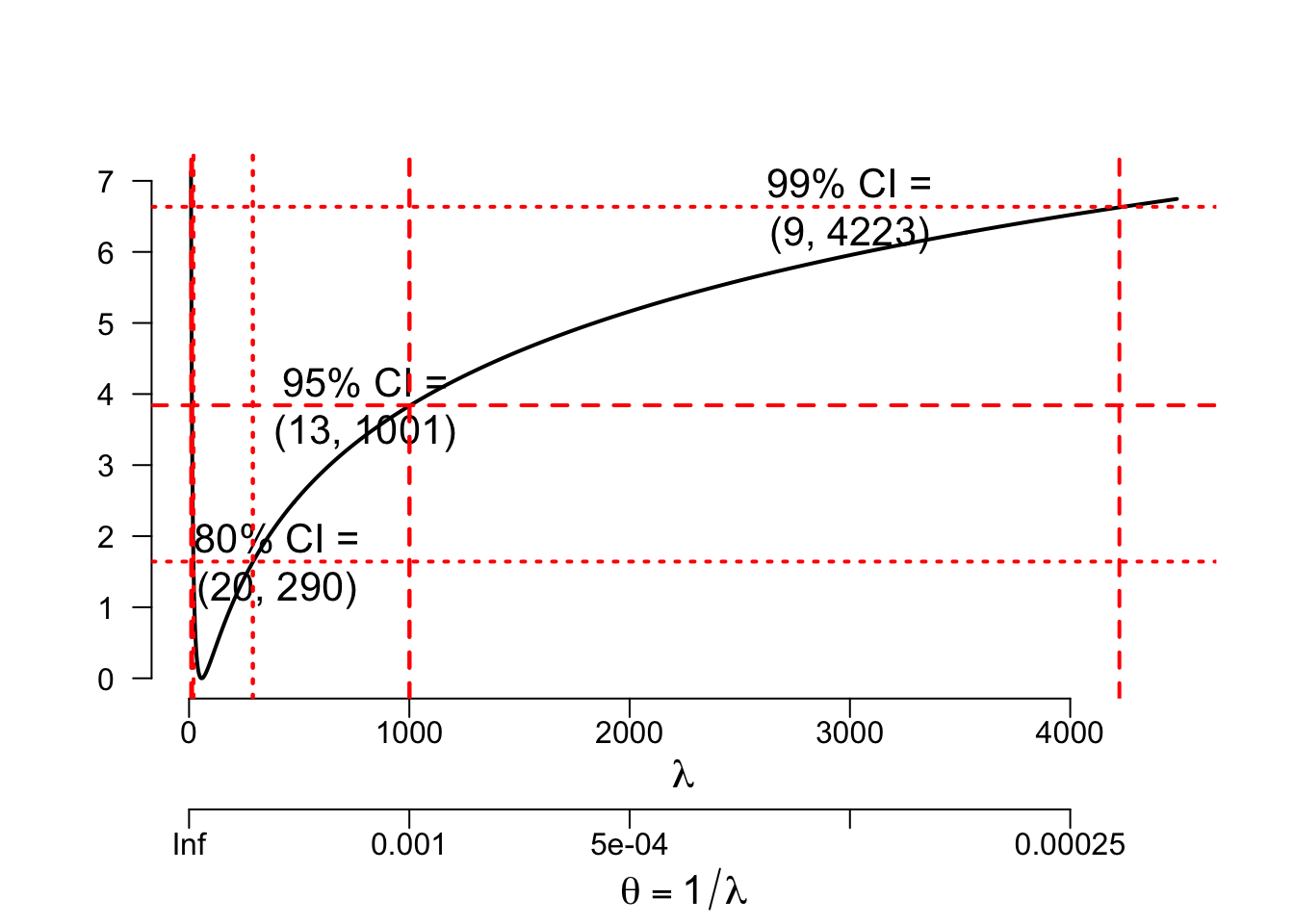## Introduction

This is a companion to the Wikiversity article on “Time to extinction of civilization”. That article assumes the 1962 Cuban Missile Crisis and the 1983 Soviet nuclear false alarm incident provide one observation on the time between major nuclear crises, with a second time between such crises being censored at the present.

What can we say about the distribution of the time between such major nuclear crises?

With one observed time and a second censored, we can construct a likelihood function, which we can then use to estimate the mean time between such crises and the uncertainty in that estimate. With further estimates of the probability that such a crisis would lead to a nuclear war and nuclear winter, we can simulate such times and obtain plausible bounds on uncertainty in our estimates.

This methodology could later be expanded to consider a larger list of nuclear crises with a broader range of probabilities for each crisis escalating to a nuclear war and winter. The fact that no such nuclear war has occurred as of this writing puts an upper limit on such probabilities. A rough lower limit can be estimated from comments from people like Robert McNamara and Daniel Ellsberg, both of whom have said that as long as there are large nuclear arsenals on earth, it is only a matter of time before a nuclear crises escalates to such a nuclear Armageddon. McNamara was US Secretary of Defense during the 1962 Cuban Missile Crisis, and Ellsberg as a leading nuclear war planner advising McNamara and the rest of President Kennedy’s team during that crisis. For more on this, see the companion Wikiversity article on “Time to extinction of civilization”.

We start by being explicit about the observed and censored times between major nuclear crises.

## Times of major nuclear crises

str(eventDates <- c(as.Date(
c('1962-10-16', '1983-09-26')), Sys.Date()))
##  Date[1:3], format: "1962-10-16" "1983-09-26" "2020-02-03"
(daysBetween <- difftime(tail(eventDates, -1),
head(eventDates, -1), units='days'))
## Time differences in days
##   7650 13279
(yearsBetween <- as.numeric(daysBetween)/365.24)
##  20.94513 36.35692
names(yearsBetween) <- c('observed', 'censored')
str(yearsBetween)
##  Named num [1:2] 20.9 36.4
##  - attr(*, "names")= chr [1:2] "observed" "censored"

## Likelihood of times between major nuclear crises

Appendix 1 of that Wikiversity article provides the following likelihood assuming we observe times between major nuclear crises, $$T_1, ..., T_{k-1},$$ plus one censoring time, $$T_k$$, and the times between such crises follow an exponential distribution that does not change over time:

$L(\lambda | \mathbf{T}) = \exp[−S_k / \lambda ] / \lambda^{k-1}$

where $$\mathbf{T}$$ = the vector consisting of $$T_1, ..., T_k$$, and

$S_k = \sum_{i=1}^k{T_i}.$

The exponential distribution is the simplest lifetime distribution. It is widely used for applications like this and seems reasonable in this context.

[For setting math in RMarkdown, we are following Cosma Shalizi (2016) “Using R Markdown for Class Reports”.]

We code this as follows:

Lik <- function(lambda, Times=yearsBetween){
Lik <- (exp(-sum(Times)/lambda) /
(lambda^(length(Times)-1)))
Lik
}

From this, we compute the log(likelihood) as follows:

$l(\lambda | \mathbf{T}) = [(−S_k / \lambda) - (k-1)\log(\lambda)].$

We code this as follows:

logLk <- function(lambda, Times=yearsBetween){
logL <- (-sum(Times)/lambda -
(length(Times)-1)*log(lambda))
logL
}

By differentiating $$l$$ with respect to $$\lambda$$ or $$u = \log(\lambda)$$ or $$\theta = 1/\lambda$$, we get a score function that is zero when $$\lambda$$ is $$\sum T_i/(k-1)$$, where the “-1” comes from assuming that only the last of Times is censored.

The value of parameter(s) that maximize the likelihood is (are) called maximum likelihood estimates (MLEs), and it is standard to distinguish an MLE with a circumflex (^). We use this convention to write the following:

$\hat\lambda = \sum T_i / (k-1).$

This is commonly read “lambda hat”. We code it as follows:

(lambdaHat <- (sum(yearsBetween) /
(length(yearsBetween)-1)))
##  57.30205

From Wilks’ theorem, we know that 2*log(likelihood ratio) is approximately Chi-squared with degrees of freedom equal to the number of parameters estimated, which is 1 in this case.

#(chisq2 <- qchisq(
#             c(.8, .95, .99, .999, 1-1e-6), 1))
(chisq2 <- qchisq(c(.2, .05, .01, .001, 1e-6),
1, lower.tail=FALSE))
##   1.642374  3.841459  6.634897 10.827566 23.928127

In reality, because of the questionable nature of our assumptions, we may wish to place less confidence in these numbers than what is implied by the stated confidence levels. However, we will not change these numbers but leave it to the reader to downgrade them as seems appropriate.

Also, in the following, we will mark the 80, 95, and 99 percent confidence intervals on the plots, leaving the more extreme tails for separate computations. For now, we want to plot 2*log(likelihood) in a neighborhood of the MLE. For this, we will focus on the region that is closer than chisq2/2 of the maximum:

lambda <- lambdaHat+seq(-50, 1000, 50)
(logLR2 <- 2*(logLk(lambdaHat) - logLk(lambda)))
##   9.5744251 0.0000000 0.3226741 0.7482237 1.1245174 1.4492355 1.7319259
##   1.9812388 2.2038111 2.4046247 2.5874494 2.7551829 2.9100900 3.0539699
##  3.1882728 3.3141837 3.4326824 3.5445878 3.6505912 3.7512809 3.8471622
##  3.9386716

After several attempts at adjusting the parameters for the seq function while including 0 in the sequence, I gave up trying to get a range with 0 inside and just over qchisq(0.99, 1) = 6.63 on both ends: Obviously, I got it for $$\lambda$$ small. However, it seemed infeasible to do this with only a relatively few points for $$\lambda$$ large: The MLE here is only the second of 22 evenly-spaced points on the $$\lambda$$ scale while the value for the 22nd point is not close to the target 6.63. Let’s try $$u = \log(\lambda)$$:

l_lam <- log(lambdaHat)+seq(-2, 6, 1)
(logLR2_u <- 2*(logLk(lambdaHat) - logLk(exp(l_lam))))
##   8.7781122  1.4365637  0.0000000  0.7357589  2.2706706  4.0995741  6.0366313
##   8.0134759 10.0049575

This seems more sensible, though still somewhat skewed, with the MLE as the third of 9 evenly-spaced points on the $$u$$ scale. What about $$\theta = 1/\lambda$$?

theta <- (1/lambdaHat + seq(-.016, .06, .004))
(logLR2_th <- 2*(logLk(lambdaHat) - logLk(1/theta)))
##   3.14013816 0.95184991 0.30968285 0.06225756 0.00000000 0.04567595
##   0.16213041 0.32860522 0.53231709 0.76483246 1.02029363 1.29446601
##  1.58418884 1.88704025 2.20112350 2.52492536 2.85721918 3.19699685
##  3.54341973 3.89578273

This looks worse: With $$\hat\theta = 1/\hat\lambda = 0.0178$$, it seems infeasible to get a sequence of only a few equally-spaced positive numbers that include 0 and still produce numbers just over qchisq(0.99, 1) = 6.63 on either ends, as we created on the $$\lambda$$ scale, let alone both as we have on the $$u$$ scale.

This suggests we should parameterize our analysis in terms of $$u = \log(\lambda)$$. To confirm this, let’s create plots on all three scales, starting with $$u$$.

library(grDevices)
outType <- ''
#outType = 'png'

switch(outType,
svg=svg('yrs2Armageddon.svg'),
#   need png(..., 960, 960), because the default 480
#   is not sufficiently clear to easily read the labels
png=png('yrs2Armageddon.png', 960, 960)
)
op <- par(mar=c(6, 4, 4, 2)+.1)

# Experiment with the range of "seq" here until
# head and tail of logLR2_u. are just over 6.63:
u. <- log(lambdaHat)+seq(-1.86, 4.36, .02)
lam. <- exp(u.)
logLR2_u. <- 2*(logLk(lambdaHat) - logLk(lam.))
head(logLR2_u., 1)
##  7.127474
tail(logLR2_u., 1)
##  6.745557
plot(lam., logLR2_u., type='l', bty='n', log='x',
xlab='', ylab='', las=1, axes=FALSE, lwd=2)
axis(2, las=1)

# xlab = \lambda:
# Greek letters did not render in GIMP 2.10.8 on 2018-12-30,
# so don't use svg until this is fixed.
switch(outType,
# cex doesn't work properly with svg > GIMP
# Therefore, I can NOT use svg
svg={cex2 <- 2; mtext('lambda', 1, 1.6, cex=cex2)},
png={cex2 <- 2; mtext(expression(lambda), 1, 1.6, cex=cex2)},
{cex2 <- 1.3; mtext(expression(lambda), 1, 1.6, cex=cex2)}
)

lamTicks <- axTicks(1)
thTicks <- 1/lamTicks
axis(1, lamTicks, thTicks, line=3, padj=-1)
switch(outType,
svg=mtext('theta == 1/lambda', 1, 4.9, cex=cex2),
mtext(expression(theta == 1/lambda), 1, 4.9, cex=cex2)
)

abline(h=chisq2, col='red', lty=c('dotted', 'dashed'),
lwd=2)
(CI.8 <- range(lam.[logLR2_u. <= chisq2]))
##   20.25368 289.55242
text(lambdaHat, chisq2,
paste0('80% CI =\n(',
paste(round(CI.8), collapse=', '), ')'),
cex=cex2)

(CI.95 <- range(lam.[logLR2_u. <= chisq2]))
##    13.04411 1000.58125
text(lambdaHat, chisq2,
paste0('95% CI =\n(',
paste(round(CI.95), collapse=', '), ')'),
cex=cex2)
abline(v=CI.8, col='red', lty='dotted', lwd=2)
abline(v=CI.95, col='red', lty='dashed', lwd=2)

(CI.99 <- range(lam.[logLR2_u. <= chisq2]))
##     9.471965 4223.149114
text(lambdaHat, chisq2,
paste0('99% CI =\n(',
paste(round(CI.99), collapse=', '), ')'),
cex=cex2)

abline(v=CI.8, col='red', lty='dotted', lwd=2)
abline(v=CI.95, col='red', lty='dashed', lwd=2)
abline(v=CI.99, col='red', lty='dashed', lwd=2)if(outType != '')dev.off()

par(op)

Let’s produce this same plot without the log scale for $$\lambda$$:

# copy the code from the last snippet
# and delete "log='x'", then adjust the placement
# of CI text
switch(outType,
svg=svg('yrs2Armageddon_lin.svg'),
#   need png(..., 960, 960), because the default 480
#   is not sufficiently clear to easily read the labels
png=png('yrs2Armageddon_lin.png', 960, 960)
)
op <- par(mar=c(6, 4, 4, 2)+.1)

u. <- log(lambdaHat)+seq(-1.86, 4.36, .02)
lam. <- exp(u.)
logLR2_u. <- 2*(logLk(lambdaHat) - logLk(lam.))
head(logLR2_u., 1)
##  7.127474
tail(logLR2_u., 1)
##  6.745557
plot(lam., logLR2_u., type='l', bty='n',
xlab='', ylab='', las=1, axes=FALSE, lwd=2)
axis(2, las=1)

# xlab = \lambda:
# Greek letters did not render in GIMP 2.10.8 on 2018-12-30,
# so don't use svg until this is fixed.
switch(outType,
# cex doesn't work properly with svg > GIMP
# Therefore, I can NOT use svg
svg={cex2 <- 2; mtext('lambda', 1, 1.6, cex=cex2)},
png={cex2 <- 2; mtext(expression(lambda), 1, 1.6, cex=cex2)},
{cex2 <- 1.3; mtext(expression(lambda), 1, 1.6, cex=cex2)}
)

lamTicks <- axTicks(1)
thTicks <- 1/lamTicks
axis(1, lamTicks, thTicks, line=3, padj=-1)
switch(outType,
svg=mtext('theta == 1/lambda', 1, 4.9, cex=cex2),
mtext(expression(theta == 1/lambda), 1, 4.9, cex=cex2)
)

abline(h=chisq2, col='red', lty=c('dotted', 'dashed'),
lwd=2)
(CI.8 <- range(lam.[logLR2_u. <= chisq2]))
##   20.25368 289.55242
#text(lambdaHat, chisq2,
text(400, chisq2,
paste0('80% CI =\n(',
paste(round(CI.8), collapse=', '), ')'),
cex=cex2)

(CI.95 <- range(lam.[logLR2_u. <= chisq2]))
##    13.04411 1000.58125
#text(lambdaHat, chisq2,
text(800, chisq2,
paste0('95% CI =\n(',
paste(round(CI.95), collapse=', '), ')'),
cex=cex2)
abline(v=CI.8, col='red', lty='dotted', lwd=2)
abline(v=CI.95, col='red', lty='dashed', lwd=2)

(CI.99 <- range(lam.[logLR2_u. <= chisq2]))
##     9.471965 4223.149114
#text(lambdaHat, chisq2,
text(3000, chisq2,
paste0('99% CI =\n(',
paste(round(CI.99), collapse=', '), ')'),
cex=cex2)

abline(v=CI.8, col='red', lty='dotted', lwd=2)
abline(v=CI.95, col='red', lty='dashed', lwd=2)
abline(v=CI.99, col='red', lty='dashed', lwd=2)if(outType != '')dev.off()

par(op)

The plot vs. $$\log(\lambda)$$ is obviously skewed, but this linear plot is vastly worse.

What about linear in $$\theta = 1/\lambda$$?

switch(outType,
svg=svg('yrs2Armageddon_inverse.svg'),
png=png('yrs2Armageddon_inverse.png', 960, 960)
)
op <- par(mar=c(6, 4, 4, 2)+.1)

# This will require more changes than just deleting log='x':
u. <- log(lambdaHat)+seq(-1.86, 4.36, .02)
lam. <- exp(u.)
logLR2_u. <- 2*(logLk(lambdaHat) - logLk(lam.))
head(logLR2_u., 1)
##  7.127474
tail(logLR2_u., 1)
##  6.745557
plot(-1/lam., logLR2_u., type='l', bty='n',
xlab='', ylab='', las=1, axes=FALSE, lwd=2)

thTicks <- (-axTicks(1))
axis(1, -thTicks, abs(1/thTicks), padj=-1)
axis(2, las=1)

# xlab = \lambda:
# Greek letters did not render in GIMP 2.10.8 on 2018-12-30,
# so don't use svg until this is fixed.
switch(outType,
# cex doesn't work properly with svg > GIMP
# Therefore, I can NOT use svg
svg={cex2 <- 2; mtext('lambda', 1, 1.6, cex=cex2)},
png={cex2 <- 2; mtext(expression(lambda), 1, 1.6, cex=cex2)},
{cex2 <- 1.3; mtext(expression(lambda), 1, 1.6, cex=cex2)}
)

axis(1, -thTicks, thTicks, line=3, padj=-1)
switch(outType,
svg=mtext('theta == 1/lambda', 1, 4.9, cex=cex2),
mtext(expression(theta == 1/lambda), 1, 4.9, cex=cex2)
)

abline(h=chisq2, col='red', lty=c('dotted', 'dashed'),
lwd=2)
(CI.8 <- range(lam.[logLR2_u. <= chisq2]))
##   20.25368 289.55242
#text(lambdaHat, chisq2,
text(-.02, chisq2,
paste0('80% CI =\n(',
paste(round(CI.8), collapse=', '), ')'),
cex=cex2)

(CI.95 <- range(lam.[logLR2_u. <= chisq2]))
##    13.04411 1000.58125
#text(lambdaHat, chisq2,
text(-.04, chisq2,
paste0('95% CI =\n(',
paste(round(CI.95), collapse=', '), ')'),
cex=cex2)
abline(v=CI.8, col='red', lty='dotted', lwd=2)
abline(v=CI.95, col='red', lty='dashed', lwd=2)

(CI.99 <- range(lam.[logLR2_u. <= chisq2]))
##     9.471965 4223.149114
#text(lambdaHat, chisq2,
text(-.06, chisq2,
paste0('99% CI =\n(',
paste(round(CI.99), collapse=', '), ')'),
cex=cex2)

abline(v=-1/CI.8, col='red', lty='dotted', lwd=2)
abline(v=-1/CI.95, col='red', lty='dashed', lwd=2)
abline(v=-1/CI.99, col='red', lty='dashed', lwd=2)